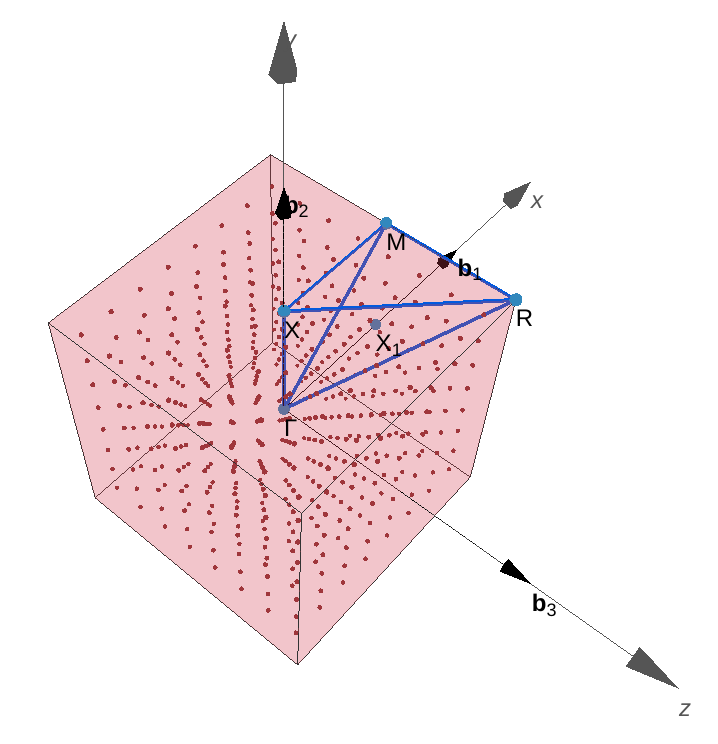# `widget-bzvisualizer`: A Jupyter widget to visualize the 1st Brillouin zone and band paths#

Source code: osscar-org/widget-bzvisualizer

Introduction: A Jupyter widget to plot the 1st Brillouin zone and band paths. It uses the Javascript package tools-seekpath developed by Materials Cloud.

## 1. Import the widget from the package#

```from widget_bzvisualizer import BZVisualizer
import numpy as np
from ase.dft.kpoints import *
```

## 2. Initialize the parameters for the widget#

• cell: the cell vectors a, b, c

• positions: the coordinates of the atoms

• elements: the elements of the atoms in an array

```lattice = np.array([[1, 0, 0], [0, 1, 0], [0, 0, 1]])

w = BZVisualizer(lattice, [[0.0, 0.0, 0.0]], , True)
display(w)
```### One can also change the kpoints coordinates in the widget#

```w.kpts = (monkhorst_pack((8, 8, 8))*2*np.pi).tolist()
```